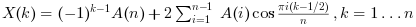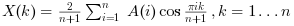Scilab Home page | Wiki | Bug tracker | Forge | Mailing list archives | ATOMS | File exchange
Scilab 6.1.0
Change language to: English - Français - 日本語 - Русский

# dst

Discrete sine transform.

# idst

Inverse discrete sine transform.

### Syntax

X=dst(A [,sign] [,option])
X=dst(A,sign,selection [,option])
X=dst(A,sign,dims,incr [,option])
X=idst(A [,option])
X=idst(A,selection [,option])
X=idst(A,dims,incr [,option])

### Arguments

A

a real or complex vector or real or complex array (vector, matrix or N-D array.

X
a real or complex array with same shape as A.
sign
an integer. with possible values 1 or -1. Select direct or inverse transform. The default value is -1 (direct transform).
selection
a vector containing index on A array dimensions. See the Description part for details.
dims
a vector of positive numbers with integer values, or a vector of positive integers. See the Description part for details.

Each element must be a divisor of the total number of elements of A.

The product of the elements must be less than the total number of elements of A.

incr
a vector of positive numbers with integer values, or a vector of positive integers. See the Description part for details.

incr must have the same number of elements than dims.

Each element must be a divisor of the total number of elements of A.

The incr elements must be in strictly increasing order.

option
a character string. with possible values "dst1", "dst2", "dst4" or "dst" for direct transform and "dst1", "dst3", "dst4" or "idst" for inverse transform. The default value is "dst" for direct transform and "idst" for inverse transform. See the Description part for details.

### Transform description

This function realizes direct or inverse 1-D or N-D Discrete Sine Transforms with shift depending on the option parameter value:

• For "dst1" the function computes the unnormalized DST-I transform. The 1-D transform of a vector of length n is:• For "dst2" the function computes the unnormalized DST-II transform. the 1-D transform of a vector of length n is:• For "dst3" the function computes the unnormalized DST-III transform. The 1-D transform of a vector of length n is:• For "dst4" the function computes the unnormalized DST-IV transform. the 1-D transform of a vector of length n is:• For "dst" the function computes the normalized DST-I transform. The 1-D transform of a vector of length n is:• For "idst" the function computes the normalized DST-I transform. The 1-D transform of a vector of length n is:The multi-dimensional DST transforms , in general, are the separable product of the given 1d transform along each dimension of the array. For unnormalized transforms , computing the forward followed by the backward/inverse multi-dimensional transform will result in the original array scaled by the product of the dimension sizes.

### Syntax description

Short syntax
direct

X=dst(A,-1 [,option]) or X=dst(A [,option]) gives a direct transform according to the option value. The default is normalized DST-I direct transform.

If A is a vector (only one dimension greater than 1) a 1-d transform is performed and in the other cases a n-dimensional transform is done.

(the -1 argument refers to the sign of the exponent..., NOT to "inverse"),

inverse

X=dst(A,1 [,option]) or X=idst(A [,option])performs the inverse transform.

If A is a vector (only one dimension greater than 1) a 1-d transform is performed and in the other cases a n-dimensional transform is done.

Long syntax for DST along specified dimensions
• X=dst(A,sign,selection [,option]) allows to perform efficiently all direct or inverse dst of the "slices" of A along selected dimensions.

For example, if A is a 3-D array X=dst(A,-1,2) is equivalent to:

for i1=1:size(A,1),
for i3=1:size(A,3),
X(i1,:,i3)=dst(A(i1,:,i3),-1);
end
end

and X=dst(A,-1,[1 3]) is equivalent to:

for i2=1:size(A,2),
X(:,i2,:)=dst(A(:,i2,:),-1);
end
• X=dst(A,sign,dims,incr) is an old syntax that also allows to perform all direct or inverse dst of the slices of A along selected dimensions.

For example, if A is an array with n1*n2*n3 elements X=dst(A,-1,n1,1) is equivalent to X=dst(matrix(A,[n1,n2,n3]),-1,1). and X=dst(A,-1,[n1 n3],[1 n1*n2]) is equivalent to X=dst(matrix(A,[n1,n2,n3]),-1,[1,3]).

### Optimizing dst

Remark: fftw function automatically stores his last parameters in memory to re-use it in a second time. This improves greatly the time computation when consecutives calls (with same parameters) are performed.

It is possible to go further in dst optimization using get_fftw_wisdom, set_fftw_wisdom functions.

### Algorithms

This function is based on the fftw3 library.

### Bibliography

Matteo Frigo and Steven G. Johnson, "FFTW Documentation" http://www.fftw.org/#documentation